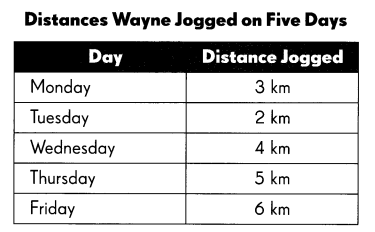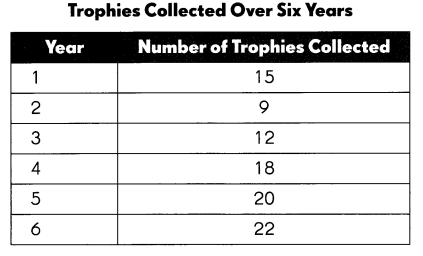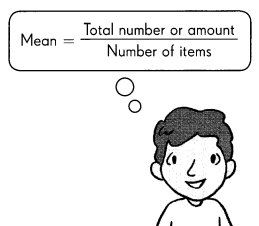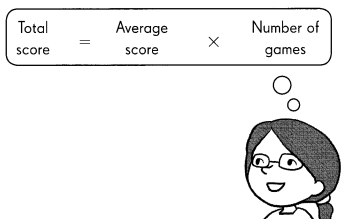# Math in Focus Grade 4 Chapter 5 Practice 1 Answer Key Average

Go through the Math in Focus Grade 4 Workbook Answer Key Chapter 5 Practice 1 Average to finish your assignments.

## Math in Focus Grade 4 Chapter 5 Practice 1 Answer Key Average

Find the mean or average of each set of data.

Example
6, 14, 18,22
Step 1
Find the sum of the four numbers.
6 + 14 + 18 + 22 = 60
Step 2
Divide the sum by 4.
60 ÷ 4 = 15The mean or average of the set of numbers is 15

Question 1.
Here are the weights of 5 pieces of luggage at an airport.
14 lb, 18 lb, 21 lb, 27 lb, 30 lb
Step 1
Find the total weight of all the pieces of luggage.
___ + ___ + ___ + ____ + ____
= ____ lb
Step 2
Divide the total by 5.
____ ÷ 5 = ___ lb
What is the average weight of the pieces of luggage? ____ lb

The total weight of all the pieces of luggage is 110 lb.
The average weight of the pieces of luggage is 22 lb.

Explanation:
Step 1:
Given that the weight of 5 pieces of luggage at an airport is 14 lb, 18 lb, 21 lb, 27 lb, 30 lb. So the total weight of all the pieces of luggage is 14 lb+18 lb+21  lb+27 lb+30 lb = 110 lb.
Step 2:
Here, we will divide the total weight of 5 pieces of luggage which is 110lb
110 lb ÷ 5 = 22 lb.
So the average weight of the pieces of luggage is 22 lb.

Find the mean of each set of data.

Question 2.
37, 0, 67, 44
The mean is 37.

Explanation:
Here, to find the mean we will add all the given data, and then we will divide by the number of values in the set. So the mean of the given data is
37+0+67+44 which is 148 ÷ 4 = 37.

Question 3.
$8,$12, $15,$29
The mean is $16. Explanation: Here, to find the mean we will add all the given data, and then we will divide by the number of values in the set. So the mean of the given data is$8+$12+$15+\$29 which is 64 ÷ 4 = 16.

Question 4.
15 pt, 21 pt, 34 pt, 48 pt, 52 pt
The mean is 34pt.

Explanation:
Here, to find the mean we will add all the given data, and then we will divide by the number of values in the set. So the mean of the given data is
15 pt+21 pt+34 pt+48 pt+52 pt which is 170 ÷ 5 = 34.

Question 5.
28yd, 61 yd, 19yd, 43yd, 89yd, 126yd
The mean is 61yd.

Explanation:
Here, to find the mean we will add all the given data, and then we will divide by the number of values in the set. So the mean of the given data is
28yd+61 yd+19yd+43yd+89yd+126yd which is 366 ÷ 6 = 61.

Question 6.
55 lb, 246 lb, 1oo lb, 34 lb, 95 lb, 460 lb
The mean is 165lb.

Explanation:
Here, to find the mean we will add all the given data, and then we will divide by the number of values in the set. So the mean of the given data is
55 lb+246 lb+1oo lb+34 lb+95 lb+460 lb which is 990 ÷ 6 = 165.

Complete. Use the data in the table.

The table shows the distances Wayne jogged on 5 days.Question 7.
How many kilometers did he jog altogether?
The number of kilometers did he jog altogether is 20 km.

Explanation:
Given that the distances Wayne jogged on 5 days, so the number of kilometers did he jog altogether is 3+2+4+5+6 which is 20 km.

Question 8.
On average, how many kilometers did he jog each day?
The average number of kilometers did he jog each day is 4 km.

Explanation:
The average number of kilometers did he jog each day is 20÷5 which is 4 km.Complete. Use the data in the table.

The table shows the number of trophies a school collected over 6 years.Question 9.
What is the total number of trophies collected in 6 years?
The total number of trophies collected in 6 years is 96.

Explanation:
Given that the number of trophies a school collected over 6 years, so the total number of trophies collected in 6 years is 15+9+12+18+20+22 which is 96.

Question 10.
What is the average number of trophies collected each year?The average number of trophies collected each year is 96.

Explanation:
The average number of trophies collected each year is 96÷6 which is 16.

Example
Mrs. Lim made 6,250 milliliters of orange juice and poured it into 5 containers. Find the mean amount of juice in each container.
6,250 ÷ 5 = 1,250 mL
The mean amount of a juice in each container is 1,250 mL.Question 11.
A chess club began accepting members on January 1. By September 30 of the same year, the club had a total of
504 members. What was the average number of members who joined the club each month?
The average number of members who joined the club each month is 56 members.

Explanation:
Given that a chess club began accepting members on January 1 and by September 30 of the same year, the club had a total of 504 members. As there are 9 months from January to September,  so the average number of members who joined the club each month is 504÷30 which is 56 members.

Example
The average number of goals scored by a soccer team in a game was 4. The team played a total of 22 games. What was the total number of goals scored by the team?
4 × 22 = 88 goalsQuestion 12.
The mean length of the sides of a triangular plot of land is 18 yards. What is its perimeter?
The mean length of the sides of the triangular plot of land is 54 yards.

Explanation:
Given that the perimeter of the triangular plot is 18 yards. As the formula of the perimeter is
(a+b+c)/3. So the mean length of the sides of the triangular plot of land is (a+b+c)/3 = 18 which is
a+b+c = 18×3
= 54 yards.

Question 13.
There are 12 peaches in a carton. The mean mass of all the peaches is 175 grams. What is their total mass?